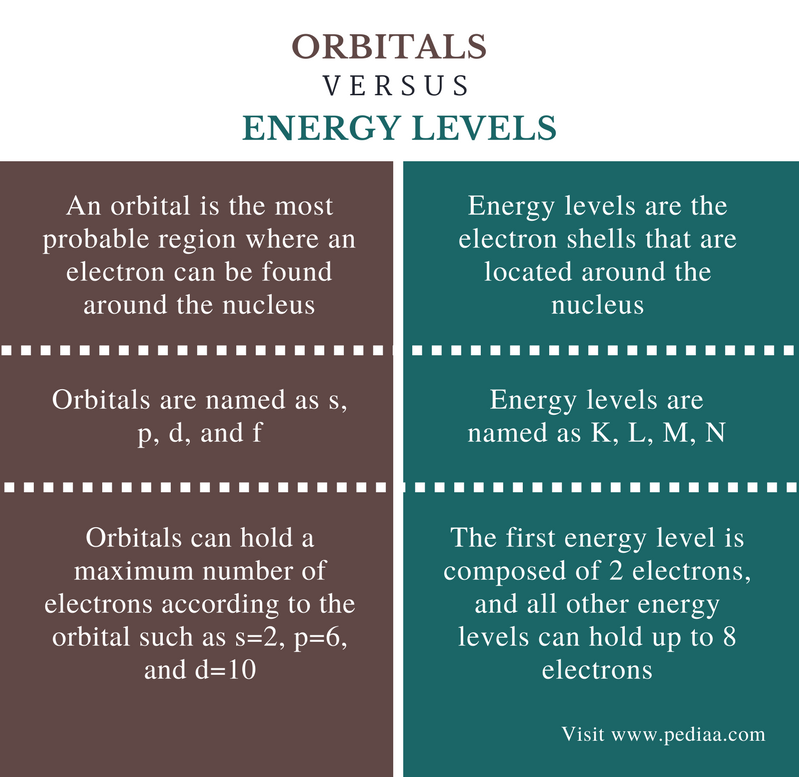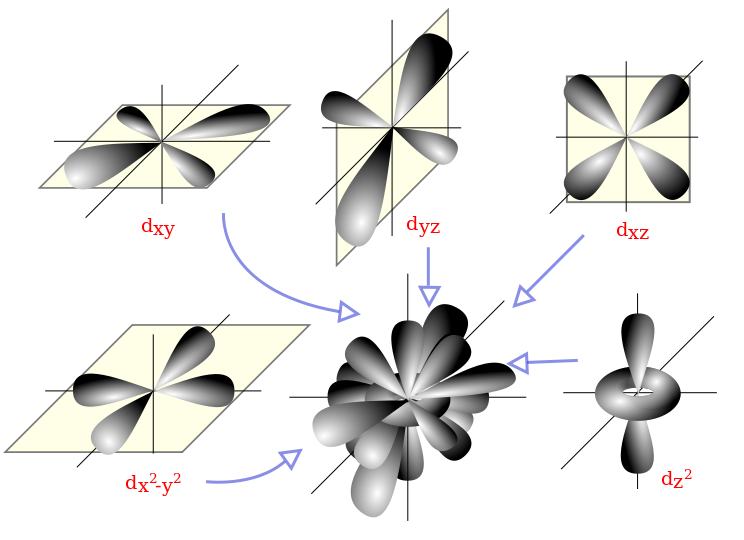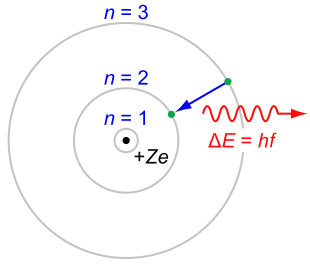Difference Between Orbitals and Energy Levels

Main Difference – Orbitals vs Energy Levels

Each and every atom is composed of a nucleus that is made out of protons and neutrons that are surrounded by electrons. These electrons are in continuous movement around the nucleus. Therefore, we cannot give a specific location for an electron in an atom. Instead of locating the exact position of an electron, scientists have introduced the concept of “probability.” In other words, the most probable pathway that an electron is most likely to be moving is determined. This pathway is called an orbital. These orbitals are arranged according to the amount of energy that electrons in these orbitals are composed of. These are called energy levels. The main difference between orbitals and energy levels is that orbitals show the most probable pathway of an electron that is in motion around the nucleus whereas energy levels show the relative locations of orbitals according to the amount of energy they possess.

Key Areas Covered

1. What are Orbitals
– Formation, Properties, and Arrangement
2. What are Energy Levels
– Formation, Properties, and Arrangement
3. What is the Relationship Between Orbitals and Energy Levels
– Orbitals and Energy Levels
4. What is the Difference Between Orbitals and Energy Levels
– Comparison of Key Differences

Key Terms: Atom, d Orbital, Electron, Energy Levels, Orbitals, Probability, p Orbital, s OrbitalWhat are Orbitals

An orbital can be defined as the most probable region where an electron can be found around the nucleus. In the atomic level, the most accurate name for an orbital is atomic orbital. The atomic orbital may exist in several shapes such as spherical shape and dumbbell shape. Orbital indicates the most probable pathway of an electron that is in motion around the nucleus of an atom.

There are several types of orbitals that can be found around the nucleus. Few of them are described below.

s orbital

These are spherical-shaped orbitals. In the same energy level, s orbitals have the lowest energy. The maximum number of electrons that an s orbital can hold is two. These two electrons are in opposite spin so that the repulsion between two electrons is minimized.

p orbital

These are dumbbell shaped orbitals having a higher energy than s orbital. The maximum number of electrons that p orbital can hold is 6. This is because one p orbital is composed of three sub orbitals named as px, py and pz. Each of these orbitals can hold a maximum of 2 electrons.

d orbital

These orbitals look like two dumbbells in the same plane. However, it is a complicated 3D structure than s and p orbitals. One d orbital is composed of 5 sub orbitals. Each suborbital can hold up to 2 electrons. Therefore, the maximum number of electrons that a d orbital can hold is 10.Figure 1: Shapes of atomic orbitals

According to the molecular orbital theory, when two atomic orbitals are overlapped, a molecular orbital is formed. This molecular orbital indicates the formation of a covalent bond. Therefore, orbitals are directly involved in chemical bonding.

What are Energy Levels

The electron shells that are located around the nucleus are called energy levels. These shells have discrete energy values. Electrons fill these energy levels or shells. These energy levels are named as K, L, M, N, etc. The energy level that has the lowest energy is K. Electrons are filled to these energy levels according to the ascending order of energy. In other words, the electrons are first filled to the lowest energy level. That way, the atoms can get stabilized.

There are fixed numbers of electrons that each energy level can hold. These numbers are given below. This number depends on the number of orbitals that each energy level is comprised of.

1st energy level – 2

2nd energy level – 8

3rd energy level – 8

4th energy level – 8

This indicates that all other energy levels except for the 1st energy level can hold up to 8 electrons.Figure 2: Energy levels in an atom. The symbol “n” indicates the energy level.

Electrons can move between these energy levels either by absorbing or releasing energy. When energy is given to an atom, an electron in a lower energy level can be moved to a higher energy level. This new state is called excited state. However, this excited state is not stable. Therefore, this electron may come back to the ground level by releasing energy. These processes are called electron transitions.

Relationship Between Orbitals and Energy Levels

• Orbitals of an atom are arranged according to their energies. Therefore, energy levels are composed of orbitals.

Difference Between Orbitals and Energy Levels

Definition

Orbitals: An orbital is the most probable region where an electron can be found around the nucleus.

Energy Levels: Energy levels are the electron shells that are located around the nucleus.

Naming

Orbitals: Orbitals are named as s, p, d, and f.

Energy Levels:  Energy levels are named as K, L, M, N.

Number of Electrons

Orbitals: Orbitals can hold a maximum number of electrons according to the orbital such as s=2, p=6, and d=10.

Energy Levels: The first energy level is composed of 2 electrons, and all other energy levels can hold up to 8 electrons.

Conclusion

Orbitals are composed of electrons. Energy levels show the arrangement of orbitals around an atom according to the energy of those orbitals. The main difference between orbitals and energy levels is that orbitals show the most probable pathway of an electron that is in motion around the nucleus whereas energy levels show the relative locations of orbitals according to the amount of energy that they have.

Image Courtesy:

1. “D orbitals” By T User: Sven(CC BY-SA 3.0) via Commons Wikimedia
2. “Bohr-atom-PAR” By JabberWok at the English language Wikipedia (CC BY-SA 3.0) via Commons Wikimedia